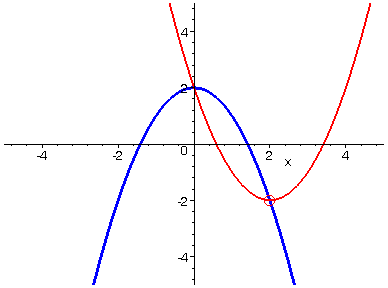VERTEX ANIMATION

A long time ago in another state of existence (Texas), I was playing around with parabolas when I noticed something unexpected. If you take a quadratic function of the form f(x) = a*x^2 + b*x + c and if you let the value of b vary over an interval, then the vertices of the resulting parabolas seem to trace out the path of yet another parabola. With a little algebra, one can show that the graph of this new parabola is given by the function y = c - a*x^2. Below is an example of what the graph of the function f(x) = x^2 + b*x + 2 looks like as b varies from -4 to 4. As the red parabola travels, you can see its vertex following the path of the function
y = 2 - x^2.

> with(plots):

> P:=animate(x^2+t*x+2, x=-5..5, t=-4..4, view=[-5..5, -5..5], thickness=2, color=red):

> Q:=plot(2-x^2, x=-5..5, view=[-5..5, -5..5], thickness=3, color=blue):

> R:=display(seq(pointplot([2-4*i/15,2-(2-4*i/15)^2],symbol=circle,symbolsize=20,color=red),i=0..15), insequence=true):

> display([P,Q,R]);>